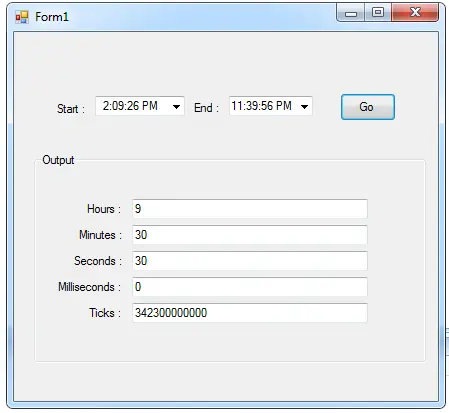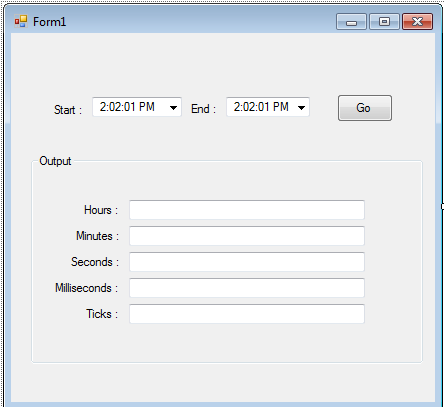# Getting Time Interval Using C#

## Getting Time Interval Using C#

Today, I’m going to teach you how to get a time interval using C#.NET. In this project, I use the TimeSpan properties to get the time interval between two Times. I also segregate the hours, minutes, seconds, millisecond and even the tick of the clock. For the result, it will appear in each corresponding text boxes.### So let’s begin:

Open Microsoft Visual Studio, create a new Project in C#  then add a new Windows Form Application. Do the Form just like this.After that, go to the solution explorer and click the view code.

In the code view, create a method to segregate the time intervals into the corresponding fields.

```private void TimeInterval(TimeSpan ts )
{

//'USE THE PROPERTIES OF THE TIMESPAN AND IT DEMONSTRATE TimeSpan.Hours
//', TimeSpan.Milliseconds , TimeSpan.Minutes , TimeSpan.Seconds
//' AND TimeSpan.Ticks
txtHr.Text = ts.Hours.ToString();
txtMin.Text = ts.Minutes.ToString();
txtSec.Text = ts.Seconds.ToString();
txtMilli.Text = ts.Milliseconds.ToString();
txtTicks.Text = ts.Ticks.ToString();
}```

Then, go back to the design view, double click the button and do the following codes in the method.

```private void button1_Click(object sender, EventArgs e)
{
//'CREATE A VARIABLE OF A TIMESPAN
TimeSpan time_span;
//'CREATE THE VARIABLE OF A DATETIME
DateTime strt_Date;
DateTime end_Date;

//'SET AND CONVERT THE TIME FROM THE DATETIMEPICKER
strt_Date = DateTime.Parse(dtpstrTime.Text);
end_Date = DateTime.Parse(dtpEndtime.Text);
//'SUBTRACT THE STARTING TIME AND THE END TIME
//time_span = end_Date.Subtract(strt_Date).Duration;

time_span = end_Date.Subtract (strt_Date).Duration();
//'PERFORM THE SUB PROCEDURE THAT YOU HAVE
//'CREATED IN DISPLAYING THE TIME INTERVAL
TimeInterval(time_span);
}```

Finally, do this following code on the first load. This will  set the value of the end time greater than the starting time.

```private void Form1_Load(object sender, EventArgs e)
{
////'SET THE VALUE OF THE END DATE HIGHER THAN THE START DATE